# Complex Fibonacci Numbers Part 1: Real Inputs

## The Fibonacci sequence:

The Fibonacci sequence is one of the most well known sequences of numbers: 1,1,2,3,5,8... Most people also know how to generate it: starting with 1 and 1, take the the last two terms and add them. Starting out, you would go 1+1=2, 1+2=3, 2+3=5, as far out as you wanted to go. But how, for example, would you find the 1000th Fibonacci number. You could do the iterative 'add the last two terms' approach 1000 times, but that would be tedious and full of errors. Well, as it turns out, there is actually a better way: Binet's formula.

## Binet's Formula:

Binet's formula is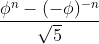where ϕ (phi) is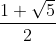(approximately 1.618), and n is the index of the Fibonacci number you want to find (n=1 is the first, n=2 is the second, etc.). The result is the nth Fibonacci number.
Try it out! Plugging in 1 for n gives 1, and plugging in 6 gives 8, as expected. But this formula also raises more questions. What happens when you ask for the 1.5th Fibonacci number? You might notice that in Binet's formula, n is a power, and when raising a number to a fractional power, you actually end up taking a root (ie.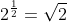). But in the second part of the formula,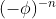, the base of the root is a negative number. That raises another question- what exactly is the root of a negative number?

## Imaginary Numbers:

I'm assuming most of the people here know what imaginary numbers are, but if you don't, Khan Academy's videos on them explain them much better than I (ha ha) could.

## Plotting

So, going back to our last question, what happens when you ask for the 1.5th Fibonacci number. Well plugging in 1.5 to the formula gives us 0.920-0.217i.And we can plot that point on the complex plane. What if we keep doing that, plugging in numbers into Binet's formula, then plotting them on the complex plane. If we do, a really interesting shape emerges:

. As you can see, it starts at 0, then enters the world of the complex numbers, before crossing the real axis at n=1, since 1 is the 1st Fibonacci number. But 1 is also the 2nd number, so it spirals around and crosses the real axis at 1 again when n=2, before crossing back and forth across the real axis whenever a "normal" Fibonacci number occurs.

## Conclusion:

These kinds of beautiful formulas and visualizations are what I love most about math. It's really amazing that the function "knows" that it needs to go through 1 twice and does that interesting spiral. I really enjoyed learning about Binet's formula and how you can get complex Fibonacci numbers, and I hope someone else out there thinks these are neat as well.

Here is a project I made that graphs Binet's formula in the complex plane.

Sorry for the wall of text...

Things get even more interesting when you plug in complex numbers to Binet's formula...

I do. I remember that complex numbers were part of the curriculum when I was in high school but this is something I don't remember. So, thank you for sharing all this.

It's a good wall of text.

The spiral is beautiful and really surprising to see.

Cool!

This is all indeed totally cool.

I had to read the actual project code to understand your graph. I thought that the horizontal axis was the input to fib, but that couldn't be right because functions can't loop back that way, by definition. But your graph doesn't show the input at all, only the output.

I want a slider for the scale of the x axis, so we can see how this function behaves for larger numbers. From the small sample shown here, the imaginary part seems to damp down quickly. A quick experiment seems to support that:

Alas, the experiment also shows that computation on irrational numbers doesn't quite get the right answer; fib of 100 should be a real integer but it has that (tiny) imaginary part.

I'd be tempted to invent a notation for nth roots containing the n and the number whose root you're taking, and extend the arithmetic functions to do exact arithmetic on them, and convert to floating point only at the moment where you're graphing a value.

(By the way, if you start with just square roots, so you'd treat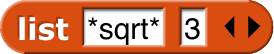as a number, the rational numbers plus their square roots form the set of all lengths constructable using straightedge and compass. :~) )

Also, round on complex numbers does not work: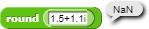I made a round block that handles that, should something like that be added to the bignums library?

Oh, interesting. Yeah, probably. @djdolphin